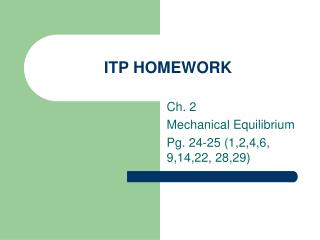Download PresentationITP HOMEWORKITP HOMEWORK - PowerPoint PPT Presentation

Download PresentationITP HOMEWORK
An Image/Link below is provided (as is) to download presentation

Download Policy: Content on the Website is provided to you AS IS for your information and personal use and may not be sold / licensed / shared on other websites without getting consent from its author. While downloading, if for some reason you are not able to download a presentation, the publisher may have deleted the file from their server.

- - - - - - - - - - - - - - - - - - - - - - - - - - - E N D - - - - - - - - - - - - - - - - - - - - - - - - - - -
Presentation Transcript

1. ITP HOMEWORK Ch. 2 Mechanical Equilibrium Pg. 24-25 (1,2,4,6, 9,14,22, 28,29)

2. 1. What is the difference between force and net force on an object. • Force is a push or a pull; net force is the combination of all forces acting on an object.

3. 2. What is the net force on a box that is being pulled to the right w/ 40 N and to the left with 30 N? • 10 N to the right 30 N 40 N

4. 4. What two quantities are necessary to determine a vector quantity? • Magnitude and direction

5. 6. Give an example of a vector and a scalar quantity. • Vector: 45 N horizontal (force), 35 m/s east (velocity) • Scalar: 5 m (distance), 10 s (time), 45 m/s (speed)

6. 9. What is the net force on an object at rest? • Zero. All forces are balanced.

7. 14. Can an object be moving and still be in (mechanical) equilibrium? • Yes. If the object moves at a constant speed in a straight line, then the net force on the object is equal to zero. • This is referred to in the book as dynamic equilibrium.

8. 22. • 22. B is the same as D, then C and then A.

9. Ch. 2 Mechanical Equilibrium 28. Agree. If a single nonzero force acts on an object, then the object will not be in mechanical equilibrium. There must be at least one more force acting on the object to cause mechanical equilibrium. 29. Scale reads half her weight.